# AP Calculus AB Practice Test 16

### Test Information10 questions20 minutes

Calculator Disallowed

1.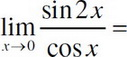2.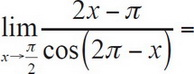3. At what point does the following function have a removable discontinuity?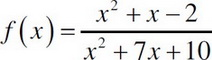4. Which of the following functions is continuous at x = 3?

5. Which of the following is the equation of the tangent line to y = 3sin2x at x =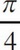?

6. What is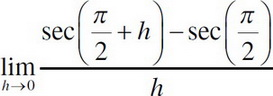?

7. If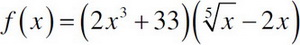, then f′(x) =

8. If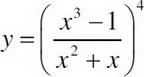, then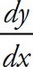=

9. Find the second derivative of x2y2 = 2 at (2, 1).

10. If the line y = ax2 + bx + c goes through the point (2, 1) and is normal to y =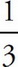x + 2 at the point (0, 2), then a = ?